Question

Q22 (Capacitors)

The figure below shows a 15.0 V battery and four uncharged capacitors of capacitances C1 = 8.00 μF, C2 = 4.00 μF, C3 = 6.00 μF, and C4 = 3.00 μF.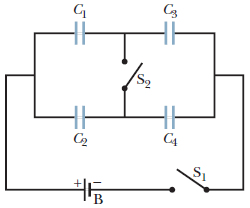If only switch S1 is closed, what is the charge on each capacitor?

(a)    C1
__________ μC

(b)    C2
__________ μC

(c)    C3
__________μC

(d)    C4
__________ μC

If both switches are closed, what is the charge on each capacitor?

(e)    C1
__________μC

(f )    C2
__________μC

(g)    C3
__________ μC

(h)    C4
__________ μC

solving for the blanks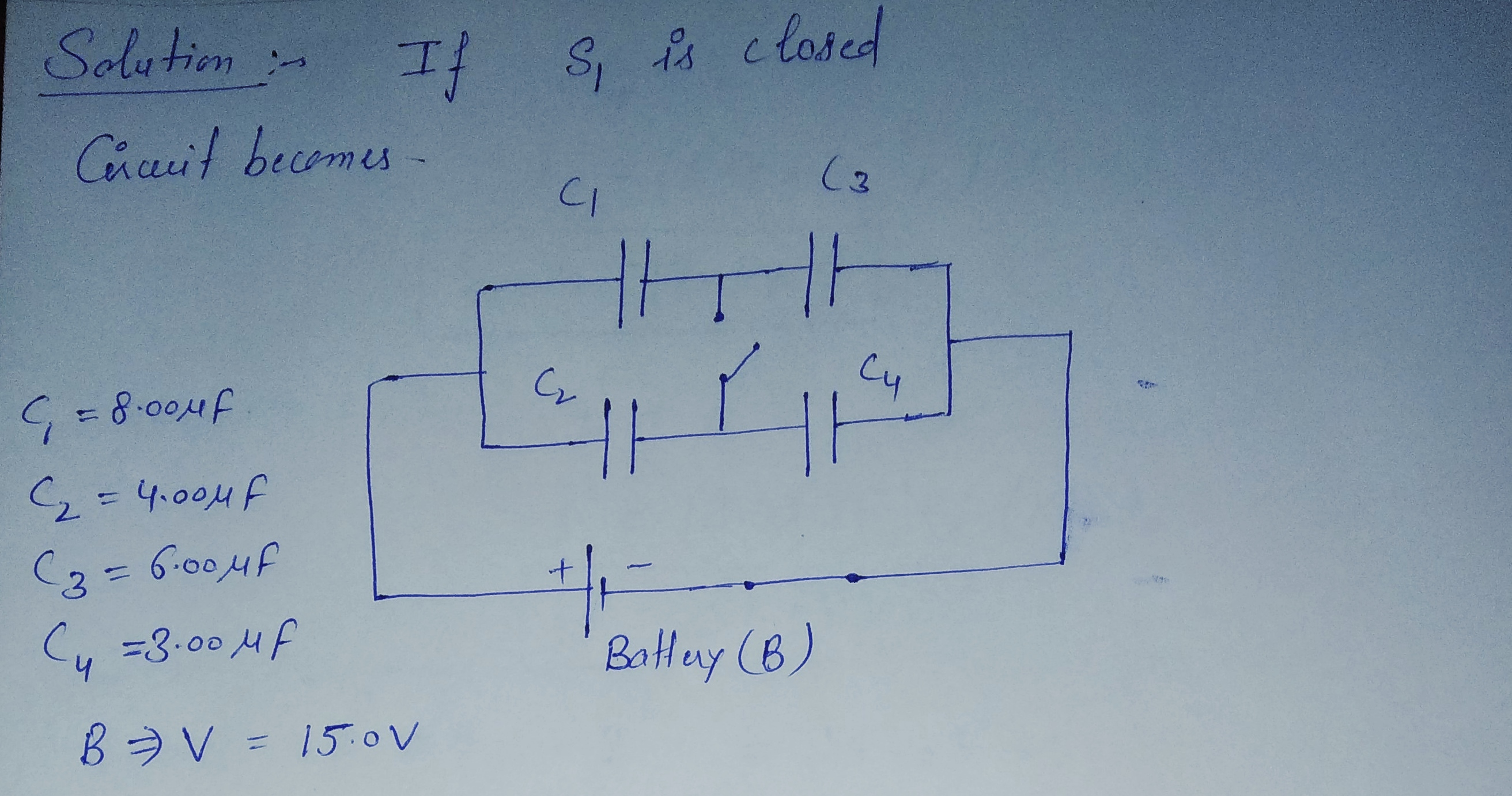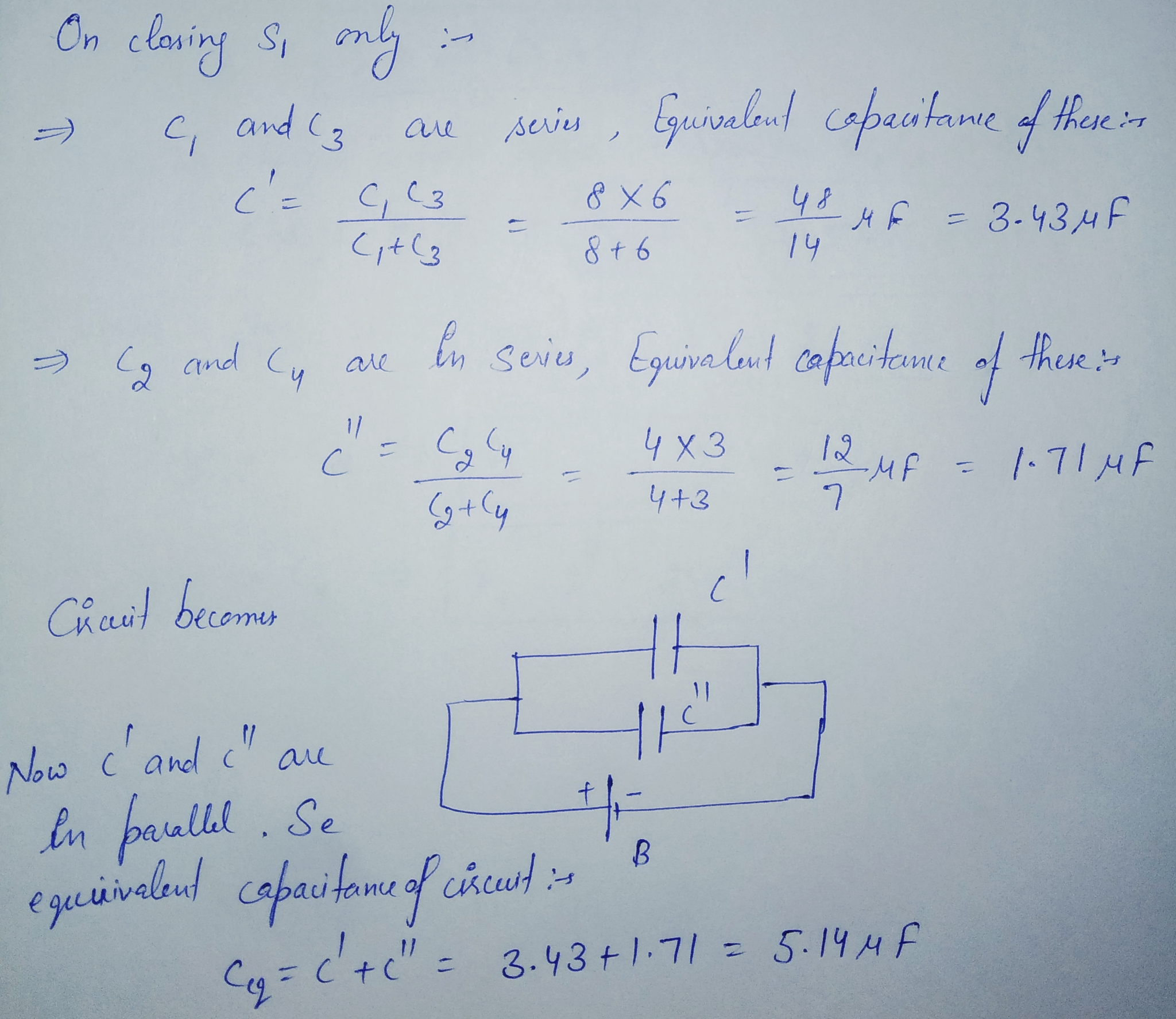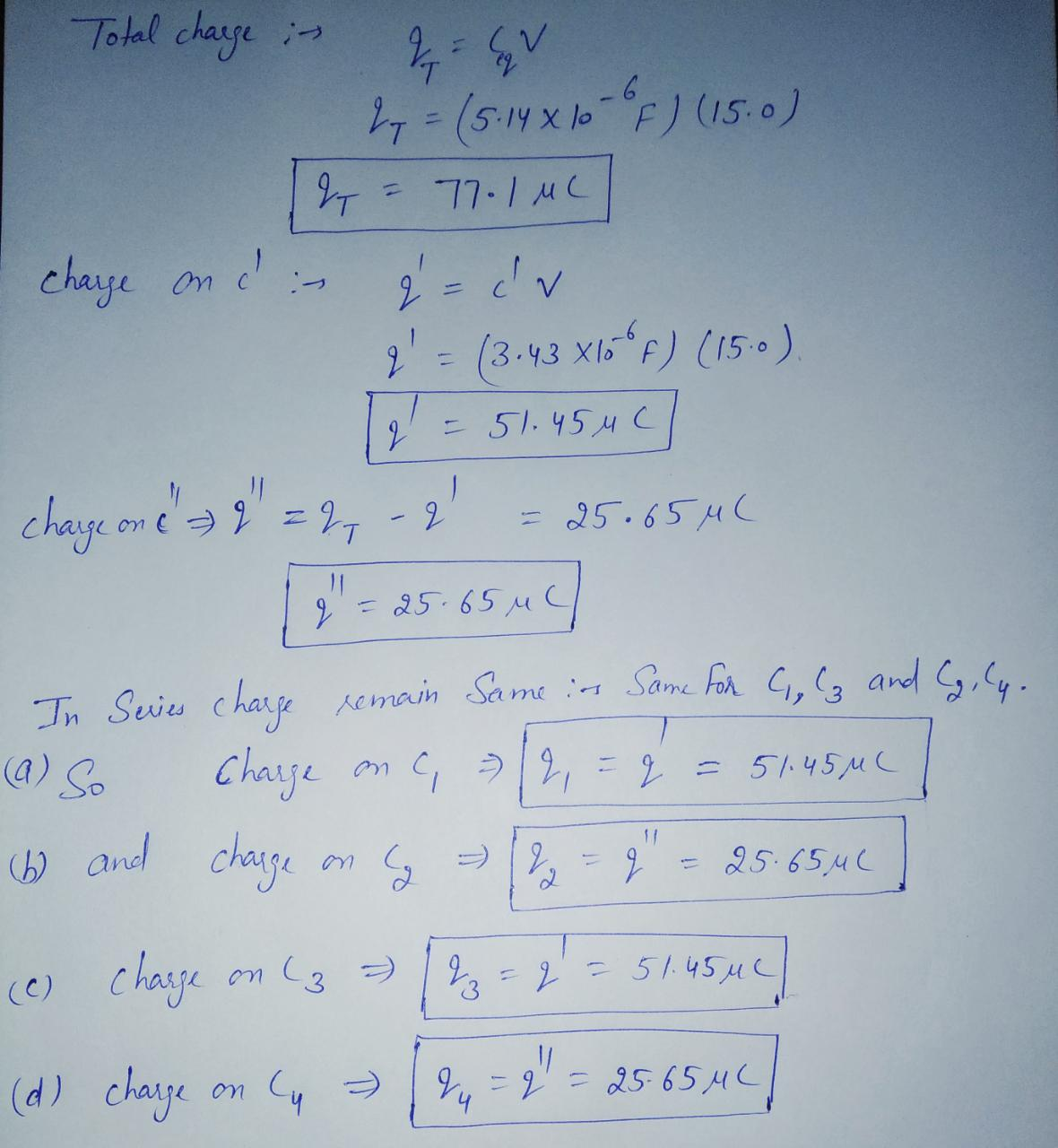#### Earn Coins

Coins can be redeemed for fabulous gifts.

Similar Homework Help Questions
• ### The figure shows a 9.18 V battery and four uncharged capacitors of capacitances C1 = 1.21...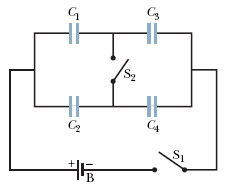The figure shows a 9.18 V battery and four uncharged capacitors of capacitances C1 = 1.21 ?F,C2 = 2.20 ?F,C3 = 3.21 ?F, and C4 = 4.25 ?F. If only switch S1 is closed, what is the charge on (a) capacitor 1, (b) capacitor 2, (c) capacitor 3, and (d) capacitor 4? If both switches are closed, what is the charge on (e) capacitor 1, (f) capacitor 2, (g) capacitor 3, and (h) capacitor 4? Cs So C2 C4

• ### The picture displays a 13.3 V battery and three uncharged capacitors of capacitances C1 = 4.47...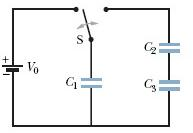The picture displays a 13.3 V battery and three uncharged capacitors of capacitances C1 = 4.47 μF,C2 = 6.48 μF and C3 = 3.48 μF. The switch is thrown to the left side until capacitor 1 is fully charged. Then the switch is thrown to the right. What is the final charge (in C) on capacitor 3?

• ### The figure displays a 12.1 V battery and three uncharged capacitors of capacitances C1 = 4.11...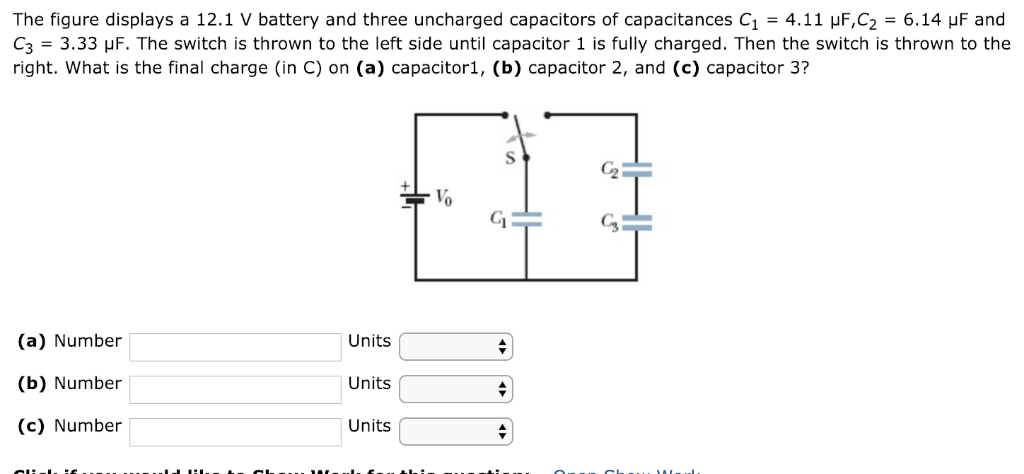The figure displays a 12.1 V battery and three uncharged capacitors of capacitances C1 = 4.11 μF,C2 = 6.14 μF and C3 = 3.33 μF. The switch is thrown to the left side until capacitor 1 is fully charged. Then the switch is thrown to the right. What is the final charge (in C) on (a) capacitor1, (b) capacitor 2, and (c) capacitor 3? Cs (a) Number (b) Number (c) Number Units Units Units

• ### The circuit in the figure below shows four capacitors connected to a battery. The switch s...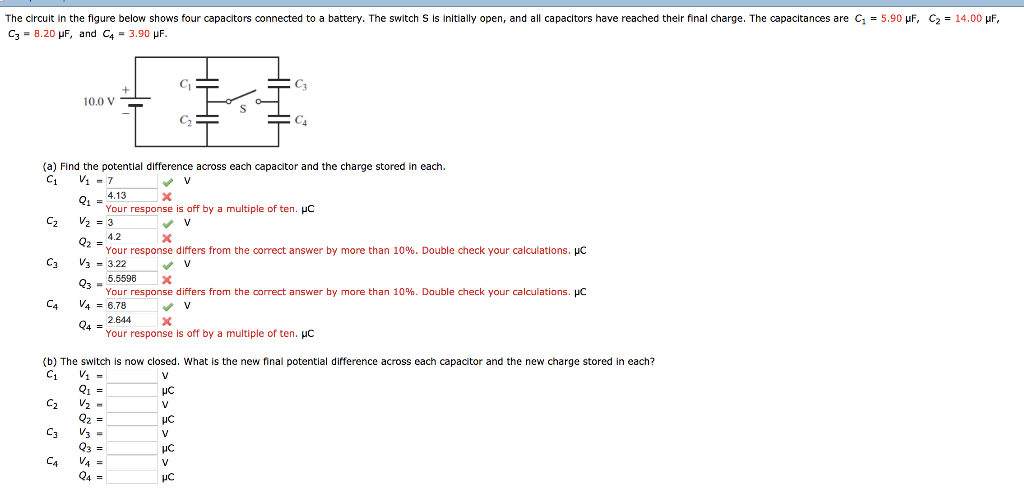The circuit in the figure below shows four capacitors connected to a battery. The switch s is initially open, and all capacitors have reached their final charge. The capacitances are C1 = 5.90 μF, C3 = 8.20 μF, and C4 = 3.90 μF C2 = 14.00 μF 10.0 V (a) Find the potential difference across each capacitor and the charge stored in each. 4.13 Your response is off by a multiple of ten. HC 4.2 Your response differs from the...

• ### 27 GO Figure 25-42 shows a 12.0 V battery and four uncharged capaci- tors of capacitances...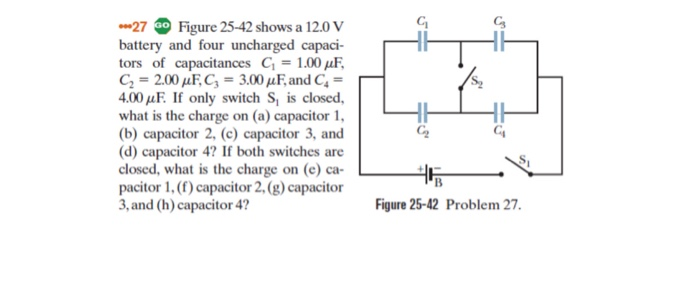27 GO Figure 25-42 shows a 12.0 V battery and four uncharged capaci- tors of capacitances G = 1.00 pF, C2 = 2.00 uF, C; = 3.00 pF, and C = 4.00 uF. If only switch S, is closed, what is the charge on (a) capacitor 1, (b) capacitor 2, (e) capacitor 3, and (d) capacitor 4? If both switches are closed, what is the charge on (e) ca- pacitor 1, (f) capacitor 2, (g) capacitor 3, and (h) capacitor...

• ### In the figure, battery B supplies 18 V. Take C1 = 1.0 μF, C2 = 2.3...

In the figure, battery B supplies 18 V. Take C1 = 1.0 μF, C2 = 2.3 μF, C3 = 3.1 μF, and C4 = 4.8 μF. Find the charge Q3 on capacitor C3 when ONLY switch S1 is closed. Find the charge Q3 on capacitor C3 when switch S2 is ALSO closed. What is the charge Q4 on capacitor C4 when switch S2 is ALSO closed?

• ### In the figure a 27 V battery is connected across capacitors of capacitances C1-C6-3.0 μF and...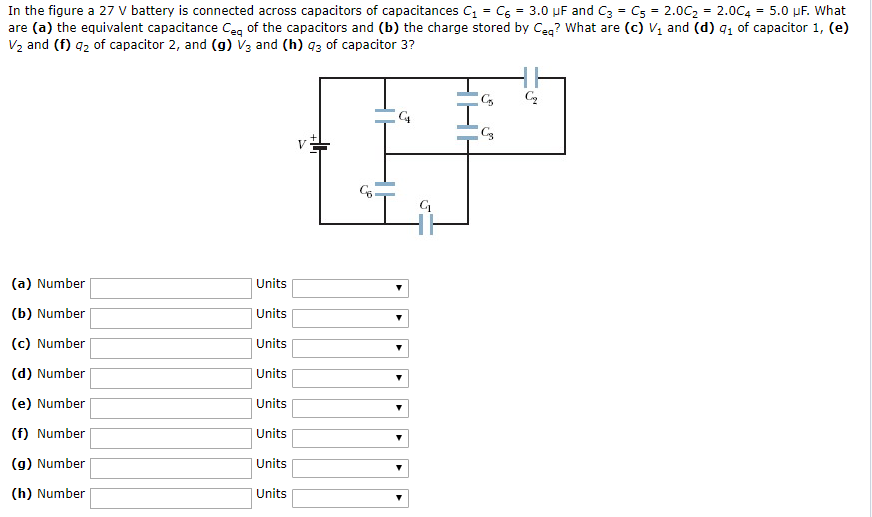In the figure a 27 V battery is connected across capacitors of capacitances C1-C6-3.0 μF and C3-Cs* 2.0C,-2.0C4-5.0 μF, what are (a) the equivalent capacitance Ceq of the capacitors and (b) the charge stored by Ceq? What are (c) V1 and (d) q1 of capacitor 1, (e) V2 and (f) 2 of capacitor 2, and (g) V3 and (h) 3 of capacitor 3? Gs C2 C4 CS C5 (a) Number (b) Number (c) Number (d) Number (e) Number (f) Number...

• ### A circuit is constructed with five capacitors and a battery as shown. The values for the...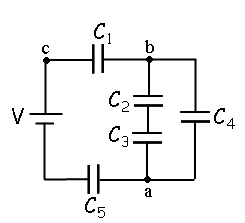A circuit is constructed with five capacitors and a battery as shown. The values for the capacitors are: C1 = C5 = 4.2 μF, C2 = 1 μF, C3 = 5.8 μF, and C4 = 4.4 μF. The battery voltage is V = 12 V. 1) What is Cab, the equivalent capacitance between points a and b?μF 2) What is Cac, the equivalent capacitance between points a and c? 3) What is Q5, the charge on capacitor C5?μC 4) What...

• ### The figure shows a circuit with an ideal battery and set of capacitors. The battery has...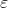The figure shows a circuit with an ideal battery and set of capacitors. The battery has an emf =12 V. The capacitors have capacitances C1 = C2 = C3 = C4 = 6 nF. (a) What is the equivalent capacitance of the entire network of capacitors? (b) What is the electrical energy stored in the capacitor with capacitance C2? We were unable to transcribe this imageProblem 6 C. The figure shows a circuit with an ideal battery and set of...

• ### In the figure a 27 V battery is connected across capacitors of capacitances C1 = C6...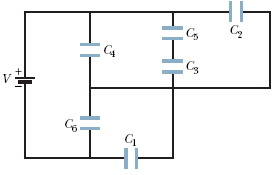In the figure a 27 V battery is connected across capacitors of capacitances C1 = C6 = 5.0 μF and C3 = C5 = 1.5C2 = 1.5C4 = 6.0 μF. What are (a) the equivalent capacitance Ceq of the capacitors and (b) the charge stored by Ceq? What are (c) V1 and (d) q1 of capacitor 1, (e) V2 and (f) q2 of capacitor 2, and (g) V3 and (h) q3 of capacitor 3? C HHH